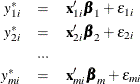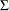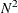# The QLIM Procedure

### Multivariate Limited Dependent Models

The multivariate model is similar to bivariate models. The generic form of the multivariate limited dependent variable model iswhere m is the number of models to be estimated. The vectorhas multivariate normal distribution with mean 0 and variance-covariance matrix. Similar to bivariate models, the likelihood may involve computing multivariate normal integrations. This is done using Monte Carlo integration. (See Genz (1992); Hajivassiliou and McFadden (1998).)

When the number of equations,, increases in a system, the number of parameters increases at the rate ofbecause of the correlation matrix. When the number of parameters is large, sometimes the optimization converges but some of the standard deviations are missing. This usually means that the model is over-parameterized. The default method for computing the covariance is to use the inverse Hessian matrix. The Hessian is computed by finite differences, and in over-parameterized cases, the inverse cannot be computed. It is recommended that you reduce the number of parameters in such cases. Sometimes using the outer product covariance matrix (COVEST=OP option) may also help.